1. 清华大学 机械工程系, 北京 100084;
2. 南方科技大学 机械与能源工程系, 深圳 518055

Simulation of the effects of shoulder-pole parameters on the waist torque during biped load walking
XU Yuning1, HUANG Jing1, CHEN Ken1, FU Chenglong21. Department of Mechanical Engineering, Tsinghua University, Beijing 100084, China;
2. Department of Mechanical and Energy Engineering, Southern University of Science and Technology, Shenzhen 518055, China
Abstract: Shoulder poles are commonly used to carry bulky loads with the weight distributed on the front and rear ends of the pole. The load transmission path reduces the torque on the waist needed to maintain the torso vertical. Traditional load carrying models have focused on the vertical direction while ignoring the influence of the waist torque to maintain the vertical alignment of the torso during walking. This paper presents a shoulder pole model for the torso. Simulations show that the waist torque is influenced by the walking speed, pole length, and suspension rope length. The simulations show that the effects of the torque on the waist should be considered while walking and longer shoulder poles, less load swing and proper position of the centroid of loads can reduce the waist torque. In practice, the shoulder pole length and suspension rope length should be chosen and the load swing should be controlled by hands to reduce the maximum torque on the waist and the energy consumption of the torque on the waist during walking.
Key words: shoulder pole model     load walking     waist torque     energy consumption

1 扁担负重行走模型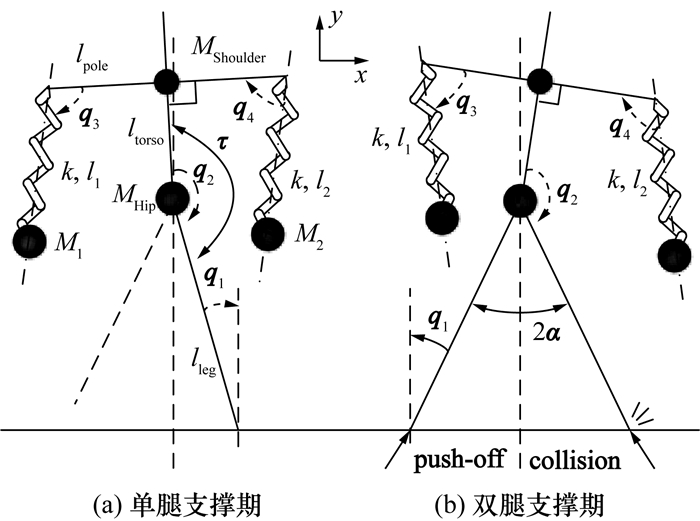图 1 重物自由摆动模型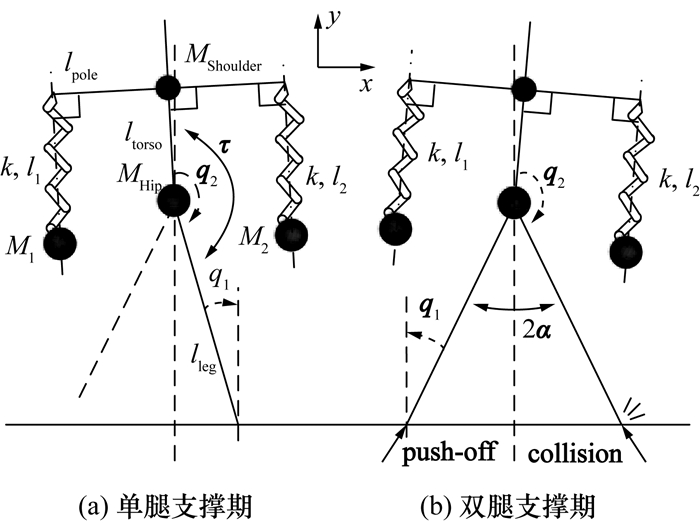图 2 重物垂直振动模型

1.1 重物自由摆动模型

1.2 重物垂直振动模型

1.3 模型参数设定

 参数名称 参数符号 参数说明 单位 设定值 腿长 lleg 代表腿的轻质刚性杆的长度；模型中地面接触点到髋部质点的距离 m 0.9 躯干长度 ltorso 代表躯干的轻质刚性杆的长度；模型中髋部质点到肩部质点的距离 m 0.46 扁担长度 lpole 代表扁担杆长度；模型中垂直于躯干杆的轻质刚性横杆的长度 m 1.3 悬绳长度 l0 代表悬挂重物的绳子长度；模型中为横杆两端弹簧的原长 m 0.3 劲度系数 k 代表扁担及悬绳的弹性；模型中为横杆两端弹簧的劲度系数 N/m 1 500 髋部质点 MHip 位于髋部的质点；模型中人体质量的主要部分 kg 55 肩部质点 MShoulder 位于肩部的质点；模型中用于等效产生躯干绕髋关节的转动惯量 kg 5 重物1 M1 位于身体后方的重物质量(质点) kg 30 重物2 M2 位于身体前方的重物质量(质点) kg 30 最大半步角 |α| 行走中腿与竖直方向的最大夹角；模型中用于判断单腿支撑期进入双腿支撑期的时机 rad 0.35

 $\mathit{\boldsymbol{\tau }} = - P(\mathit{\boldsymbol{\pi }} + {\mathit{\boldsymbol{q}}_1} - {\mathit{\boldsymbol{q}}_2}) - D\cdot{\mathit{\boldsymbol{\dot q}}_2}.$ (1)

2 模型仿真 2.1 模型仿真步骤

q(t)为t时刻的广义坐标矢量，由对应模型对应时刻的自由度组成。在重物自由摆动模型中，q(t)=[q1, q2, q3, q4, l1, l2]；在重物垂直振动模型中，q(t)=[q1, q2, l1, l2]。

 $\mathit{\boldsymbol{q}}\left( 0 \right) = {\mathit{\boldsymbol{q}}^ + }\left( t \right),$ (2)
 $\mathit{\boldsymbol{\dot q}}\left( 0 \right) = {{\mathit{\boldsymbol{\dot q}}}^ + }\left( t \right).$ (3)

2.2 评价指标及其计算方法

 ${\tau _{{\rm{max}}}} = {\rm{max}}\left( {\left| {\mathit{\boldsymbol{\tau }}\left( t \right)} \right|} \right).$ (4)

 ${E_{{\bf{push - off}}}} = {E^ + } - {E^ - }.$ (5)

 ${E_\mathit{\boldsymbol{\tau }}} = \smallint \left| {\mathit{\boldsymbol{\tau }}{\rm{d}}{\mathit{\boldsymbol{q}}_2}} \right|.$ (6)

 $\text{COT}=\frac{{消耗能量}}{{系统重量×移动距离}}.$ (7)

 ${\rm{CO}}{{\rm{T}}_{{\mathbf{push - off}}}} = \frac{{{E_{{\mathbf{push - off}}}}}}{{2g{l_{{\rm{leg}}}}{\rm{sin}}\left| \mathit{\boldsymbol{\alpha }} \right|({M_{{\rm{Hip}}}} + {M_{{\rm{Shoulder}}}} + {M_1} + {M_2})}},$ (8)
 ${\rm{CO}}{{\rm{T}}_\mathit{\boldsymbol{\tau }}} = \frac{{{E_\mathit{\boldsymbol{\tau }}}}}{{2g{l_{{\rm{leg}}}}{\rm{sin}}\left| \mathit{\boldsymbol{\alpha }} \right|\left( {{M_{{\rm{Hip}}}} + {M_{{\rm{Shoulder}}}} + {M_1} + {M_2}} \right)}}.$ (9)

3 仿真实验与结果 3.1 腰部力矩做功占总功的比例

 模型 COTτ COTpush-off COTtot=COTτ+COTpush-off (COTτ/COTtot)/% 重物自由摆动模型 0.002 781 0.019 611 0.022 392 12.4 重物垂直振动模型 0.001 453 0.015 787 0.017 240 8.4

3.2 扁担长度变化对模型的影响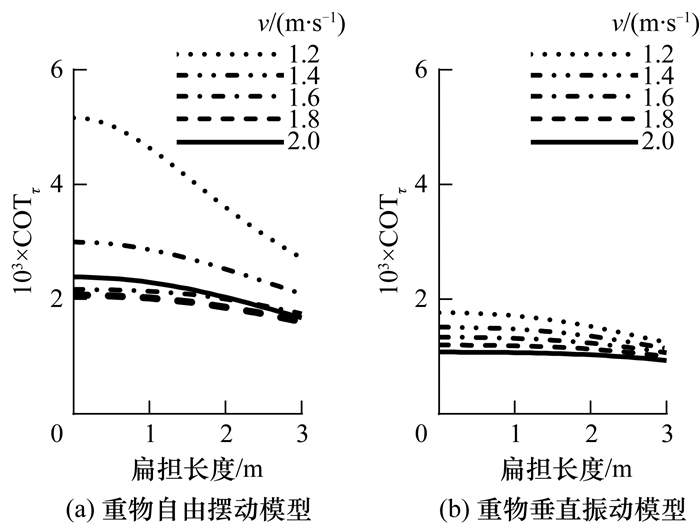图 3 扁担长度变化对COTτ的影响图 4 扁担长度变化对τmax的影响

3.3 悬绳长度变化对模型的影响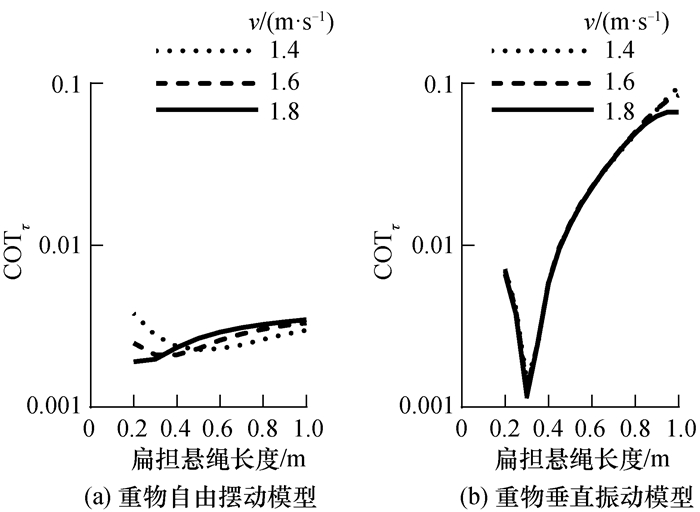图 5 扁担悬绳长度变化对COTτ的影响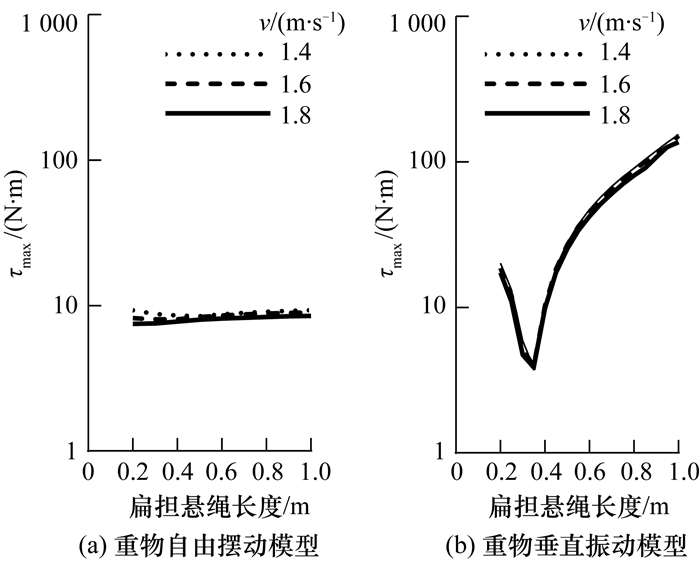图 6 扁担悬绳长度变化对τmax的影响

l0=0.3 m时，无论COTτ还是τmax，重物自由摆动模型的对应值均大于重物垂直振动模型。因此，限制重物摆动有助于节省能耗，提升负重上限。

3.4 讨论

4 总结与展望

  POTWAR K, ACKERMAN J, SEIPEL J. Design of compliant bamboo poles for carrying loads[J]. Journal of Mechanical Design, 2015, 137(1): 011404. DOI:10.1115/1.4028757  KRAM R. Carrying loads with springy poles[J]. Journal of Applied Physiology, 1991, 71(3): 1119-1122. DOI:10.1152/jappl.1991.71.3.1119  BALOGUN J A. Ergonomic comparison of three modes of load carriage[J]. International Archives of Occupational and Environmental Health, 1986, 58(1): 35-46. DOI:10.1007/BF00378538  KENNTNER G. Customs and efficiency in carrying loads of the inhabitants of the southern Himalayas. A contribution to biogeographic research[J]. Zeitschrift für Morphologie und Anthropologie, 1969, 61(2): 125-169.  BASTIEN G J, SCHEPENS B, WILLEMS P A, et al. Energetics of load carrying in Nepalese porters[J]. Science, 2005, 308(5729): 1755. DOI:10.1126/science.1111513  张义同, 张岚. 关于扁担的力学[J]. 力学与实践, 2002, 24(5): 76-78. ZHANG Y T, ZHANG L. Mechanics on the shoulder pole[J]. Mechanics in Engineering, 2002, 24(5): 76-78. DOI:10.3969/j.issn.1000-0879.2002.05.026 (in Chinese)  BIRRELL S A, HOOPER R H, HASLAM R A. The effect of military load carriage on ground reaction forces[J]. Gait & Posture, 2007, 26(4): 611-614.  邱信明. 扁担是否真的省力?[J]. 力学与实践, 2018, 40(1): 108-111. QIU X M. Is the shoulder-pole really effective?[J]. Mechanics in Engineering, 2018, 40(1): 108-111. (in Chinese)  潘晓慧, 罗元辉. 论扁担固有频率与挑夫行进频率关系[J]. 西南师范大学学报(自然科学版), 2010, 35(6): 193-195. PAN X H, LUO Y H. On relationship between inherent frequency of carrying pole and marching frequency of porter[J]. Journal of Southwest China Normal University (Natural Science Edition), 2010, 35(6): 193-195. (in Chinese)  CASTILLO E R, LIEBERMAN G M, MCCARTY L S, et al. Effects of pole compliance and step frequency on the biomechanics and economy of pole carrying during human walking[J]. Journal of Applied Physiology, 2014, 117(5): 507-517. DOI:10.1152/japplphysiol.00119.2014  ACKERMAN J, SEIPEL J. A model of human walking energetics with an elastically-suspended load[J]. Journal of Biomechanics, 2014, 47(8): 1922-1927. DOI:10.1016/j.jbiomech.2014.03.016  尤明庆. 关于扁担挑运力学原理的注记[J]. 力学与实践, 2011, 33(4): 87-89. YOU M Q. A note to the mechanical principle in carrying with shoulder-pole[J]. Mechanics in Engineering, 2011, 33(4): 87-89. (in Chinese)  WU Y H, CHEN K, FU C L. Effects of load connection form on efficiency and kinetics of biped walking[J]. Journal of Mechanisms and Robotics, 2016, 8(6): 061015. DOI:10.1115/1.4034464  GARCIA M, CHATTERJEE A, RUINA A, et al. The simplest walking model:Stability, complexity, and scaling[J]. Journal of Biomechanical Engineering, 1998, 120(2): 281-288. DOI:10.1115/1.2798313  KUO A D. Energetics of actively powered locomotion using the simplest walking model[J]. Journal of Biomechanical Engineering, 2002, 124(1): 113-120.  呼慧敏, 晁储芝, 赵朝义. 中国成年人人体尺寸数据相关性研究[J]. 人类工效学, 2014, 20(3): 49-53. HU H M, CHAO C Z, ZHAO C Y. Research on the correlation of human body size data in Chinese adults[J]. Chinese Journal of Ergonomics, 2014, 20(3): 49-53. (in Chinese)  马广韬, 孙海义, 徐玉梅. 人体尺寸回归方程的修正及应用[J]. 沈阳建筑大学学报(自然科学版), 2010, 26(3): 581-585. MA G T, SUN H Y, XU Y M. Research and application of ergonomics based on statistical method[J]. Journal of Shenyang Jianzhu University (Natural Science), 2010, 26(3): 581-585. (in Chinese)  刘静民, 仰红慧. 人体转动惯量的研究综述[J]. 体育科学, 2001, 21(4): 81-86. LIU J M, YANG H H. An overview of research on human rotational inertia[J]. Sport Science, 2001, 21(4): 81-86. (in Chinese)  SCHROEDER R T, CROFT J L, NGO G D, et al. Properties of traditional bamboo carrying poles have implications for user interactions[J]. PLoS One, 2018, 13(5): e0196208. DOI:10.1371/journal.pone.0196208